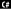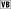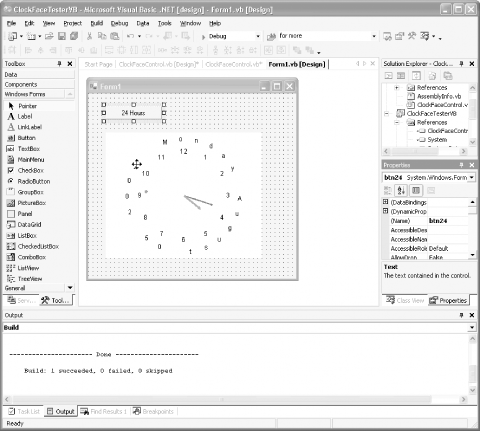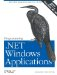# Creating Custom Controls from Scratch

Sooner or later, the Betty Crocker cookies just aren't exactly what you had in mind, and it is time to bake up a new control from scratch. In the previous examples, you derived first from an existing control type (e.g., Button) and then from UserControl. In the next example, you will derive from the base control type: Control.

Creating your control from scratch lets you manage the look and feel of your new control with precision. However, it also requires that you implement every aspect of the control, including painting itthat is, you get power, but at the cost of greater responsibility, a lesson to us all.

You'll create a control that provides the analog clock functionality you implemented in Chapter 10, but makes that functionality available to any program as a control as easy to use as a button.

Begin by creating a new Windows Control Library project in Visual Studio .NET in your language of choice. Call it ClockFaceControl. When you first create the project, you'll be put into the design mode. Right-click on the form and choose View Code. Change the name of the source file from UserControl1.cs or .vb to ClockFaceControl.cs or .vb

Next, change the name of the class from UserControl1 to ClockFaceCtrl, and change the base class from UserControl to Control.```public class ClockFaceCtrl : System.Windows.Forms.Control
``````Public Class ClockFaceCtrl
Inherits System.Windows.Forms.Control```

After you make this change to the base class, go back to the designer. You'll find that there is no longer a visible form. The Control class does not provide the form functionality that UserControl provides, but this is fine; you'll add the UI explicitly in the OnPaint method.

The code for this control is nearly identical to that shown in Chapter 10. Here are the changes:

• The ClockFaceControl derives from Control rather than Form.
• None of the form code (e.g., Dispose or InitializeComponent) is needed.
• Remove the mouse-down event handler (the control will not respond to this event). Thus, there is no need for the xCenter and yCenter member variables, and the center is set to the width and height divided by 2 and then left alone.
• Expose TwentyFourHours as a Boolean property so the client can set a 24- or 12- hour format.

You can create the ClockFaceControl by lifting the code from Chapter 10 and dropping it into a new Control class. The complete source for the control is shown in Example 17-5 and Example 17-6.

Example 17-5. The ClockFace control (C#)```using System;
using System.Collections;
using System.Drawing;
using System.Drawing.Drawing2D; // for LineCap enumerations
using System.Timers; // for onTimer event
using System.Windows.Forms;

namespace ClockFace
{

public class ClockFaceCtrl : System.Windows.Forms.Control
{
private bool b24Hours = false;
private DateTime currentTime;
private static int DateRadius = 900;
private static int offset = 0;
Font font = new Font("Arial", 80);
private StringDraw sdToday;

public ClockFaceCtrl( )
{

BackColor = SystemColors.Window;
ForeColor = SystemColors.WindowText;

string today = System.DateTime.Now.ToLongDateString( );
today = " " + today.Replace(",","");
sdToday = new StringDraw(today,this);

currentTime = DateTime.Now;

System.Timers.Timer timer = new System.Timers.Timer( );
timer.Elapsed +=
new System.Timers.ElapsedEventHandler(OnTimer);
timer.Interval = 50;
timer.Enabled = true;

}

public bool TwentyFourHours
{
get { return b24Hours; }
set { b24Hours = value; }
}

public void OnTimer(Object source, ElapsedEventArgs e)
{

Graphics g = this.CreateGraphics( );
SetScale(g);
DrawFace(g);
DrawTime(g,false);
DrawDate(g);
g.Dispose( );
}

protected override void OnPaint ( PaintEventArgs e )
{
base.OnPaint(e);
Graphics g = e.Graphics;
SetScale(g);
DrawFace(g);
DrawTime(g,true);
}

private void SetScale(Graphics g)
{
if ( Width = = 0 || Height = = 0 )
return;

// set the origin at the center
g.TranslateTransform(Width / 2, Height / 2);
// set inches to the minimum of the
// width or height divided
// by the dots per inch
float inches = Math.Min(Width / g.DpiX, Height / g.DpiX);

g.ScaleTransform(inches * g.DpiX / 2000,
inches * g.DpiY / 2000);
}

private static float GetSin(float degAngle)
{
return (float) Math.Sin(Math.PI * degAngle / 180f);
}

private static float GetCos(float degAngle)
{
return (float) Math.Cos(Math.PI * degAngle / 180f);
}

private void DrawFace(Graphics g)
{

Brush brush = new SolidBrush(ForeColor);
Brush greenBrush = new SolidBrush(Color.Green);

float x, y;

int numHours = b24Hours ? 24 : 12;
int deg = 360 / numHours;

for (int i = 1; i <= numHours; i++)
{
SizeF stringSize = g.MeasureString(
i.ToString( ),font);
x = GetCos(i*deg + 90) * FaceRadius;
y = GetSin(i*deg + 90) * FaceRadius;

StringFormat format = new StringFormat( );
format.Alignment = StringAlignment.Center;
format.LineAlignment = StringAlignment.Center;

if ( currentTime.Second = = i * 5)
g.DrawString(i.ToString( ), font,
greenBrush, -x, -y,format);
else
g.DrawString(i.ToString( ), font, brush, -x,
-y,format);
}
}

private void DrawTime(Graphics g, bool forceDraw)
{

float hourLength = FaceRadius * 0.5f;
float minuteLength = FaceRadius * 0.7f;
float secondLength = FaceRadius * 0.9f;

Pen hourPen = new Pen(BackColor);
Pen minutePen = new Pen(BackColor);
Pen secondPen = new Pen(BackColor);

hourPen.EndCap = LineCap.ArrowAnchor;
minutePen.EndCap = LineCap.ArrowAnchor;

hourPen.Width = 30;
minutePen.Width = 20;

Brush secondBrush = new SolidBrush(Color.Green);
Brush blankBrush = new SolidBrush(BackColor);

float rotation;
GraphicsState state;

DateTime newTime = DateTime.Now;
bool newMin = false;

if ( newTime.Minute != currentTime.Minute )
newMin = true;

rotation = GetSecondRotation( );
state = g.Save( );
g.RotateTransform(rotation);
g.FillEllipse(blankBrush,-25,-secondLength,50,50);
g.Restore(state);

if ( newMin || forceDraw )
{
rotation = GetMinuteRotation( );
state = g.Save( );
g.RotateTransform(rotation);
g.DrawLine(minutePen,0,0,0,-minuteLength);
g.Restore(state);

rotation = GetHourRotation( );
state = g.Save( );
g.RotateTransform(rotation);
g.DrawLine(hourPen,0,0,0,-hourLength);
g.Restore(state);
}

currentTime = newTime;

hourPen.Color = Color.Red;
minutePen.Color = Color.Blue;
secondPen.Color = Color.Green;

state = g.Save( );
rotation = GetSecondRotation( );
g.RotateTransform(rotation);
g.FillEllipse(secondBrush,-25,-secondLength,50,50);
g.Restore(state);

if ( newMin || forceDraw )
{

state = g.Save( );
rotation = GetMinuteRotation( );
g.RotateTransform(rotation);
g.DrawLine(minutePen,0,0,0,-minuteLength);
g.Restore(state);

state = g.Save( );
rotation = GetHourRotation( );
g.RotateTransform(rotation);
g.DrawLine(hourPen,0,0,0,-hourLength);
g.Restore(state);
}
}

// determine the rotation to draw the hour hand
private float GetHourRotation( )
{
// degrees depend on 24 vs. 12 hour clock
float deg = b24Hours ? 15 : 30;
float numHours = b24Hours ? 24 : 12;
return( 360f * currentTime.Hour / numHours +
deg * currentTime.Minute / 60f);
}

private float GetMinuteRotation( )
{
return( 360f * currentTime.Minute / 60f ); //+
// 6f * currentTime.Second / 60f);
}

private float GetSecondRotation( )
{
return(360f * currentTime.Second / 60f);

}

private class LtrDraw
{
char myChar;
float x;
float y;
float oldx;
float oldy;

public LtrDraw(char c)
{
myChar = c;
}

public float X
{
get { return x; }
set { oldx = x; x = value; }
}

public float Y
{
get { return y; }
set { oldy = y; y = value; }
}

public float GetWidth(Graphics g, Font font)
{
SizeF stringSize =
g.MeasureString(myChar.ToString( ),font);
return stringSize.Width;
}

public float GetHeight(Graphics g, Font font)
{
SizeF stringSize =
g.MeasureString(myChar.ToString( ),font);
return stringSize.Height;
}

public void DrawString(
Graphics g, Brush brush, ClockFaceCtrl ctrl)
{
// Font font = new Font("Arial", 40);
Font font = ctrl.font;
Brush blankBrush =
new SolidBrush(ctrl.BackColor);
g.DrawString(
myChar.ToString( ),font,blankBrush,oldx,oldy);
g.DrawString(myChar.ToString( ),font,brush,x,y);
}
} // close for nested class LtrDraw

private class StringDraw
{
ArrayList theString = new ArrayList( );
LtrDraw l;
ClockFaceCtrl theControl;

public StringDraw(string s, ClockFaceCtrl theControl)
{
this.theControl = theControl;
foreach (char c in s)
{
l = new LtrDraw(c);
}
}

public void DrawString(Graphics g, Brush brush)
{
int angle = 360 / theString.Count;
int counter = 0;

foreach (LtrDraw theLtr in theString)
{
float newX = GetCos(angle * counter + 90 -
float newY = GetSin(angle * counter + 90 -
theLtr.X = newX - (theLtr.GetWidth(g,theControl.font) / 2);
theLtr.Y = newY - (theLtr.GetHeight(g,theControl.font) / 2);
counter++;
theLtr.DrawString(g,brush,theControl);
}
ClockFaceCtrl.offset += 1;
}
} // close for nested class StringDraw

private void DrawDate(Graphics g)
{
Brush brush = new SolidBrush(ForeColor);
sdToday.DrawString(g,brush);
}

}
}```

Example 17-6. The ClockFace control (VB.NET)```Imports System
Imports System.Collections
Imports System.Drawing
Imports System.Drawing.Drawing2D
Imports System.Timers
Imports System.Windows.Forms

Namespace ClockFace

Public Class ClockFaceCtrl
Inherits System.Windows.Forms.Control
Private FaceRadius As Integer = 700
Private b24Hours As Boolean = False
Private currentTime As DateTime
Private Shared DateRadius As Integer = 900
Private Shared offset As Integer = 0
Private font As New font("Arial", 80)
Private sdToday As StringDraw

Public Sub New( )

BackColor = SystemColors.Window
ForeColor = SystemColors.WindowText

Dim today As String = System.DateTime.Now.ToLongDateString( )
today = " " + today.Replace(",", "")
sdToday = New StringDraw(today, Me)

currentTime = DateTime.Now
Dim timer As New System.Timers.Timer( )
timer.Interval = 50
timer.Enabled = True
End Sub 'New

Public Property TwentyFourHours( ) As Boolean
Get
Return b24Hours
End Get
Set(ByVal Value As Boolean)
b24Hours = Value
End Set
End Property

Public Sub OnTimer( _
ByVal [source] As [Object], _
ByVal e As ElapsedEventArgs)

Dim g As Graphics = Me.CreateGraphics( )
'Brush brush = new SolidBrush(ForeColor);
SetScale(g)
DrawFace(g)
DrawTime(g, False)
DrawDate(g)
g.Dispose( )
End Sub 'OnTimer
' DrawDate(g,brush);

Protected Overrides Sub OnPaint(ByVal e As PaintEventArgs)
MyBase.OnPaint(e)
Dim g As Graphics = e.Graphics
SetScale(g)
DrawFace(g)
DrawTime(g, True)
End Sub 'OnPaint

Private Sub SetScale(ByVal g As Graphics)
If Width = 0 Or Height = 0 Then
Return
End If
' set the origin at the center
g.TranslateTransform(Width / 2, Height / 2)

Dim inches As Single = _
Math.Min(Width / g.DpiX, Height / g.DpiX)

g.ScaleTransform( _
inches * g.DpiX / 2000, inches * g.DpiY / 2000)
End Sub 'SetScale

Private Shared Function _
GetSin(ByVal degAngle As Single) As Single
Return CSng(Math.Sin((Math.PI * degAngle / 180.0F)))
End Function 'GetSin

Private Shared Function _
GetCos(ByVal degAngle As Single) As Single
Return CSng(Math.Cos((Math.PI * degAngle / 180.0F)))
End Function 'GetCos

Private Sub DrawFace(ByVal g As Graphics)

Dim brush = New SolidBrush(ForeColor)
Dim greenBrush = New SolidBrush(Color.Green)

Dim x, y As Single

Dim numHours As Integer
If b24Hours Then
numHours = 24
Else
numHours = 12
End If

Dim deg As Integer = 360 / numHours

Dim i As Integer
For i = 1 To numHours
Dim stringSize As SizeF = _
g.MeasureString(i.ToString( ), font)
x = GetCos((i * deg + 90)) * FaceRadius
y = GetSin((i * deg + 90)) * FaceRadius

Dim format As New StringFormat( )
format.Alignment = StringAlignment.Center
format.LineAlignment = StringAlignment.Center

If currentTime.Second = i * 5 Then
g.DrawString(i.ToString( ), font, _
greenBrush, -x, -y, format)
Else
g.DrawString(i.ToString( ), font, _
brush, -x, -y, format)
End If
Next i
End Sub 'DrawFace

Private Sub DrawTime( _
ByVal g As Graphics, ByVal forceDraw As Boolean)

Dim hourLength As Single = FaceRadius * 0.5F
Dim minuteLength As Single = FaceRadius * 0.7F
Dim secondLength As Single = FaceRadius * 0.9F

Dim hourPen As New Pen(BackColor)
Dim minutePen As New Pen(BackColor)
Dim secondPen As New Pen(BackColor)

hourPen.EndCap = LineCap.ArrowAnchor
minutePen.EndCap = LineCap.ArrowAnchor

hourPen.Width = 30
minutePen.Width = 20

Dim secondBrush = New SolidBrush(Color.Green)
Dim blankBrush = New SolidBrush(BackColor)

Dim rotation As Single
Dim state As GraphicsState

Dim newTime As DateTime = DateTime.Now
Dim newMin As Boolean = False

If newTime.Minute <> currentTime.Minute Then
newMin = True
End If

rotation = GetSecondRotation( )
state = g.Save( )
g.RotateTransform(rotation)
g.FillEllipse(blankBrush, -25, -secondLength, 50, 50)
g.Restore(state)

If newMin Or forceDraw Then

rotation = GetMinuteRotation( )
state = g.Save( )
g.RotateTransform(rotation)
g.DrawLine(minutePen, 0, 0, 0, -minuteLength)
g.Restore(state)

rotation = GetHourRotation( )
state = g.Save( )
g.RotateTransform(rotation)
g.DrawLine(hourPen, 0, 0, 0, -hourLength)
g.Restore(state)
End If

currentTime = newTime

hourPen.Color = Color.Red
minutePen.Color = Color.Blue
secondPen.Color = Color.Green

state = g.Save( )
rotation = GetSecondRotation( )
g.RotateTransform(rotation)
g.FillEllipse(secondBrush, -25, -secondLength, 50, 50)
g.Restore(state)

If newMin Or forceDraw Then

state = g.Save( )
rotation = GetMinuteRotation( )
g.RotateTransform(rotation)
g.DrawLine(minutePen, 0, 0, 0, -minuteLength)
g.Restore(state)

state = g.Save( )
rotation = GetHourRotation( )
g.RotateTransform(rotation)
g.DrawLine(hourPen, 0, 0, 0, -hourLength)
g.Restore(state)
End If
End Sub 'DrawTime

' determine the rotation to draw the hour hand
Private Function GetHourRotation( ) As Single
' degrees depend on 24 vs. 12 hour clock
Dim deg As Single
Dim numHours As Single
If b24Hours Then
deg = 15
numHours = 24
Else
deg = 30
numHours = 12
End If

Return 360.0F * currentTime.Hour / numHours + deg * _
currentTime.Minute / 60.0F
End Function 'GetHourRotation

Private Function GetMinuteRotation( ) As Single
Return 360.0F * currentTime.Minute / 60.0F '+
End Function 'GetMinuteRotation
' 6f * currentTime.Second / 60f);

Private Function GetSecondRotation( ) As Single
Return 360.0F * currentTime.Second / 60.0F
End Function 'GetSecondRotation
_

Private Class LtrDraw
Private myChar As Char
Private _x As Single
Private _y As Single
Private oldx As Single
Private oldy As Single

Public Sub New(ByVal c As Char)
myChar = c
End Sub 'New

Public Property X( ) As Single
Get
Return _x
End Get
Set(ByVal Value As Single)
oldx = _x
_x = Value
End Set
End Property

Public Property Y( ) As Single
Get
Return _y
End Get
Set(ByVal Value As Single)
oldy = _y
_y = Value
End Set
End Property

Public Function GetWidth( _
ByVal g As Graphics, ByVal font As font) _
As Single
Dim stringSize As SizeF = _
g.MeasureString(myChar.ToString( ), font)
Return stringSize.Width
End Function 'GetWidth

Public Function GetHeight( _
ByVal g As Graphics, ByVal font As font) _
As Single
Dim stringSize As SizeF = _
g.MeasureString(myChar.ToString( ), font)
Return stringSize.Height
End Function 'GetHeight

Public Sub DrawString( _
ByVal g As Graphics, ByVal brush As Brush, _
ByVal ctrl As ClockFaceCtrl)
' Font font = new Font("Arial", 40);
Dim font As Font = ctrl.font
Dim blankBrush = New SolidBrush(ctrl.BackColor)
g.DrawString(myChar.ToString( ), font, _
blankBrush, oldx, oldy)
g.DrawString(myChar.ToString( ), _
font, brush, X, Y)
End Sub 'DrawString
End Class 'LtrDraw
_

Private Class StringDraw
Private theString As New ArrayList( )
Private l As LtrDraw
Private theControl As ClockFaceCtrl

Public Sub New(ByVal s As String, _
ByVal theControl As ClockFaceCtrl)
Me.theControl = theControl
Dim c As Char
For Each c In s
l = New LtrDraw(c)
Next c
End Sub 'New

Public Sub DrawString( _
ByVal g As Graphics, ByVal brush As Brush)
Dim angle As Integer = 360 / theString.Count
Dim counter As Integer = 0

Dim theLtr As LtrDraw
For Each theLtr In theString
Dim newX As Single = _
GetCos((angle * counter + 90 - _
ClockFaceCtrl.offset)) * _

Dim newY As Single = _
GetSin((angle * counter + 90 - _
ClockFaceCtrl.offset)) * _
theLtr.X = newX - theLtr.GetWidth( _
g, theControl.font) / 2
theLtr.Y = newY - theLtr.GetHeight( _
g, theControl.font) / 2
counter += 1
theLtr.DrawString(g, brush, theControl)
Next theLtr
ClockFaceCtrl.offset += 1
End Sub 'DrawString
End Class 'StringDraw

Private Sub DrawDate(ByVal g As Graphics)
Dim brush = New SolidBrush(ForeColor)
sdToday.DrawString(g, brush)
End Sub 'DrawDate
End Class 'ClockFaceCtrl
End Namespace 'ClockFace```

17.3.1 Testing the custom control

As you did with the UserControl, you'll test the custom control by creating a testing project in the same solution as the control. Call the new test project ClockFaceTester. Add a reference to your control, and then update the toolbar to include the control (you may need to browse to the dll for the control). Drag the control onto the form, name it clockFace, and then resize the form to fit.

Finally, drag a button onto the form, set its text to 24 Hours, and set its name to btn24. Your form should now look like Figure 17-14.

Figure 17-14. Clock control test formYou can see already that the clock control is working, but you'll want to set the 24-hour property programmatically. Double-click on the button to create its event handler:```private void btn24_Click(object sender, System.EventArgs e)
{
if ( clockFace.TwentyFourHours )
{
btn24.Text = "24 Hours";
clockFace.TwentyFourHours = false;
clockFace.Invalidate( );
}
else
{
btn24.Text = "12 Hours";
clockFace.TwentyFourHours = true;
clockFace.Invalidate( );
}
}
Private Sub btn24_Click( _
ByVal sender As System.Object, ByVal e As System.EventArgs) _
Handles btn24.Click
If clockFace.TwentyFourHours Then
btn24.Text = "24 Hours"
clockFace.TwentyFourHours = False
clockFace.Invalidate( )
Else
btn24.Text = "12 Hours"
clockFace.TwentyFourHours = True
clockFace.Invalidate( )
End If
End Sub```

That's it! Set the tester program as the startup project and run ityou'll see the control executing on the form much as it did in Chapter 10, but this time it is a custom control you can drop on any form.Programming .Net Windows Applications
ISBN: 0596003218
EAN: 2147483647
Year: 2003
Pages: 148